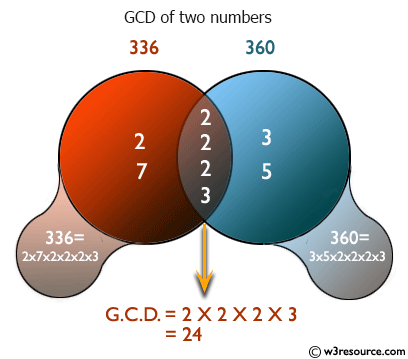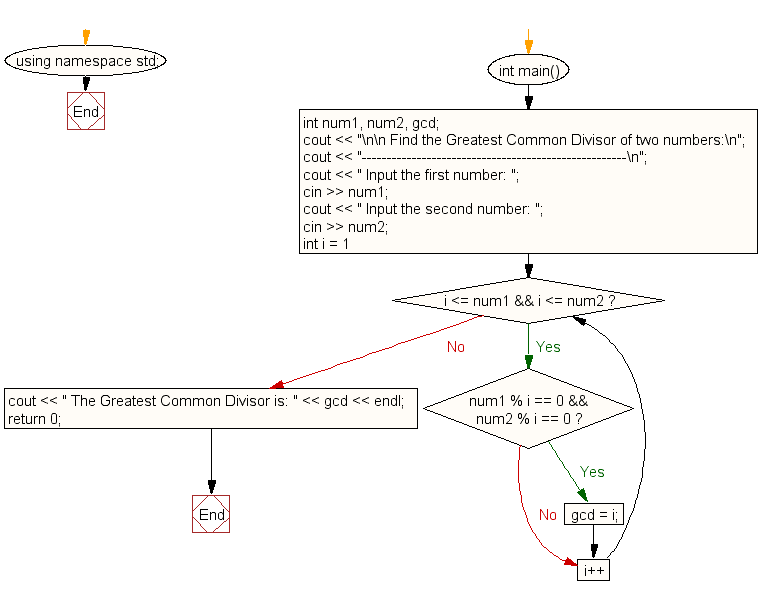﻿ C++ : Find the Greatest Common Divisor (GCD) of two numbers# C++ Exercises: Find the Greatest Common Divisor (GCD) of two numbers

## C++ For Loop: Exercise-9 with Solution

Write a program in C++ to find the Greatest Common Divisor (GCD) of two numbers.

Pictorial Presentation:Sample Solution :-

C++ Code :

``````#include <iostream>
using namespace std;
int main()
{
int num1, num2, gcd;
cout << "\n\n Find the Greatest Common Divisor of two numbers:\n";
cout << "-----------------------------------------------------\n";
cout << " Input the first number: ";
cin >> num1;
cout << " Input the second number: ";
cin >> num2;

for (int i = 1; i <= num1 && i <= num2; i++)
{
if (num1 % i == 0 && num2 % i == 0)
{
gcd = i;
}
}
cout << " The Greatest Common Divisor is: " << gcd << endl;
return 0;
}
``````

Sample Output:

``` Find the Greatest Common Divisor of two numbers:
-----------------------------------------------------
Input the first number: 25
Input the second number: 15
The Greatest Common Divisor is: 5
```

Flowchart:C++ Code Editor:

Contribute your code and comments through Disqus.

What is the difficulty level of this exercise?

﻿

## C++ Programming: Tips of the Day

What is a smart pointer and when should I use one?

This answer is rather old, and so describes what was 'good' at the time, which was smart pointers provided by the Boost library. Since C++11, the standard library has provided sufficient smart pointers types, and so you should favour the use of std::unique_ptr, std::shared_ptr and std::weak_ptr.

There was also std::auto_ptr. It was very much like a scoped pointer, except that it also had the "special" dangerous ability to be copied - which also unexpectedly transfers ownership.

It was deprecated in C++11 and removed in C++17, so you shouldn't use it.

```std::auto_ptr<MyObject> p1 (new MyObject());
std::auto_ptr<MyObject> p2 = p1; // Copy and transfer ownership.
// p1 gets set to empty!
p2->DoSomething(); // Works.
p1->DoSomething(); // Oh oh. Hopefully raises some NULL pointer exception.
```

Ref : https://bit.ly/3mc9GHE